Sections
Test Prep

# Test Prep

### Multiple Choice

#### 20.1Magnetic Fields, Field Lines, and Force

1.

For a magnet, a domain refers to ______.

1. the region between the poles of the magnet
2. the space around the magnet that is affected by the magnetic field
3. the region within the magnet in which the magnetic poles of individual atoms are aligned
4. the region from which the magnetic material is mined
2.

In the region just outside the south pole of a magnet, the magnetic field lines ______.

1. point away from the south pole
2. go around the south pole
3. are less concentrated than at the north pole
4. point toward the south pole
3.
Which equation gives the force for a charge moving through a magnetic field?
1. $F=qvBsin⁡θ$
2. $F=IℓBsin⁡θ$
3. $F=IℓB$
4. $F=qvB$
4.
Can magnetic field lines cross each other? Explain why or why not.
1. Yes, magnetic field lines can cross each other because that point of intersection indicates two possible directions of magnetic field, which is possible.
2. No, magnetic field lines cannot cross each other because that point of intersection indicates two possible directions of magnetic field, which is not possible.
5.

True or false—If a magnet shatters into many small pieces, all the pieces will have north and south poles

1. true
2. false

#### 20.2Electromagnetic Induction

6.

What does emf stand for?

1. Electromotive force
2. Electro motion force
3. Electromagnetic factor
4. Electronic magnetic factor
7.
Which formula gives magnetic flux?
1. $μ0I2πr$
2. $qvBsinθ$
3. $−NΔΦΔt$
4. $BAcos⁡θ$
8.
What is the relationship between the number of coils in a solenoid and the emf induced in it by a change in the magnetic flux through the solenoid?
1. The induced emf is inversely proportional to the number of coils in a solenoid.
2. The induced emf is directly proportional to the number of coils in a solenoid.
3. The induced emf is inversely proportional to the square of the number of coils in a solenoid.
4. The induced emf is proportional to square of the number of coils in a solenoid.
9.

True or false—If you drop a bar magnet through a copper tube, it induces an electric current in the tube.

1. false
2. true

#### 20.3Motors, Generators, and Transformers

10.

An electrical generator ________.

2. must be turned by hand
3. converts other sources of power into electrical power
4. uses magnetism to create electrons
11.

A step-up transformer increases the ________.

1. voltage from power lines for use in homes
2. current from the power lines for use in homes
3. current from the electrical generator for transmission along power lines
4. voltage from the electrical power plant for transmission along power lines
12.

What would be the effect on the torque of an electric motor of doubling the width of the current loop in the motor?

1. Torque remains the same.
2. Torque is doubled.
4. Torque is halved.
13.
Why are the coils of a transformer wrapped around a loop of ferrous material?
1. The magnetic field from the source coil is trapped and also increased in strength.
2. The magnetic field from the source coil is dispersed and also increased in strength.
3. The magnetic field from the source coil is trapped and also decreased in strength.
4. Magnetic field from the source coil is dispersed and also decreased in strength.

#### 20.1Magnetic Fields, Field Lines, and Force

14.
Given a bar magnet, a needle, a cork, and a bowl full of water, describe how to make a compass.
1. Magnetize the needle by holding it perpendicular to a bar magnet’s north pole and pierce the cork along its longitudinal axis by the needle and place the needle-cork combination in the water. The needle now orients itself along the magnetic field lines of Earth.
2. Magnetize the needle by holding it perpendicular to a bar magnet’s north pole and pierce the cork along its longitudinal axis by the needle and place the needle-cork combination in the water. The needle now orients itself perpendicular to the magnetic field lines of Earth.
3. Magnetize the needle by holding its axis parallel to the axis of a bar magnet and pierce the cork along its longitudinal axis by the needle and place the needle-cork combination in the water. The needle now orients itself along the magnetic field lines of Earth.
4. Magnetize the needle by holding its axis parallel to the axis of a bar magnet and pierce the cork along its longitudinal axis by the needle and place the needle-cork combination in the water. The needle now orients itself perpendicular to the magnetic field lines of Earth.
15.
Give two differences between electric field lines and magnetic field lines.
1. Electric field lines begin and end on opposite charges and the electric force on a charge is in the direction of field, while magnetic fields form a loop and the magnetic force on a charge is perpendicular to the field.
2. Electric field lines form a loop and the electric force on a charge is in the direction of field, while magnetic fields begin and end on opposite charge and the magnetic force on a charge is perpendicular to the field.
3. Electric field lines begin and end on opposite charges and the electric force on a charge is in the perpendicular direction of field, while magnetic fields form a loop and the magnetic force on a charge is in the direction of the field.
4. Electric field lines form a loop and the electric force on a charge is in the perpendicular direction of field, while magnetic fields begin and end on opposite charge and the magnetic force on a charge is in the direction of the field.
16.

To produce a magnetic field of 0.0020 T, what current is required in a 500-turn solenoid that is 25 cm long?

1. 0.80 A
2. 1.60 A
3. 80 A
4. 160 A
17.
You magnetize a needle by aligning it along the axis of a bar magnet and just outside the north pole of the magnet. Will the point of the needle that was closest to the bar magnet then be attracted to or repelled from the south pole of another magnet?
1. The needle will magnetize and the point of needle kept closer to the north pole will act as a south pole. Hence, it will repel the south pole of other magnet.
2. The needle will magnetize and the point of needle kept closer to the north pole will act as a south pole. Hence, it will attract the south pole of other magnet.
3. The needle will magnetize and the point of a needle kept closer to the north pole will act as a north pole. Hence, it will repel the south pole of the other magnet.
4. The needle will magnetize and the point of needle kept closer to the north pole will act as a north pole. Hence, it will attract the south pole of other magnet.
18.
Using four solenoids of the same size, describe how to orient them and in which direction the current should flow to make a magnet with two opposite-facing north poles and two opposite-facing south poles.
1.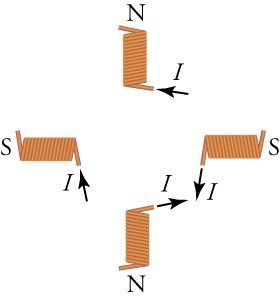2.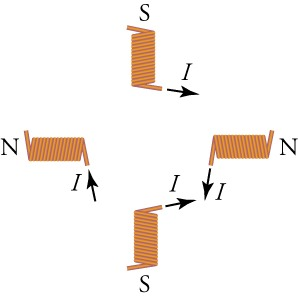3.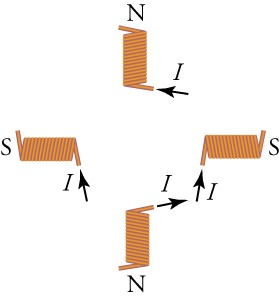4.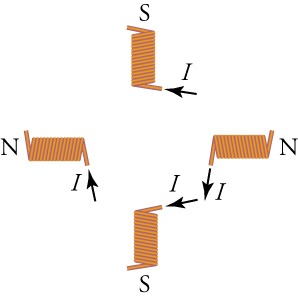19.

How far from a straight wire carrying 0.45 A is the magnetic field strength 0.040 T?

1. 0.23 µm
2. 0.72 µm
3. 2.3 µm
4. 7.2 µm

#### 20.2Electromagnetic Induction

20.
A uniform magnetic field is perpendicular to the plane of a wire loop. If the loop accelerates in the direction of the field, will a current be induced in the loop? Explain why or why not.
1. No, because magnetic flux through the loop remains constant.
2. No, because magnetic flux through the loop changes continuously.
3. Yes, because magnetic flux through the loop remains constant.
4. Yes, because magnetic flux through the loop changes continuously.
21.

The plane of a square wire circuit with side 4.0 cm long is at an angle of 45° with respect to a uniform magnetic field of 0.25 T. The wires have a resistance per unit length of 0.2. If the field drops to zero in 2.5 s, what magnitude current is induced in the square circuit?

1. 35 µA
2. 87.5 µA
3. 3.5 mA
4. 35 A
22. Yes or no—If a bar magnet moves through a wire loop as shown in the figure, is a current induced in the loop? Explain why or why not.
Figure 20.44
1. No, because the net magnetic field passing through the loop is zero.
2. No, because the net magnetic field passing through the loop is nonzero.
3. Yes, because the net magnetic field passing through the loop is zero.
4. Yes, because the net magnetic field line passing through the loop is nonzero.
23.

What is the magnetic flux through an equilateral triangle with side 60 cm long and whose plane makes a 60° angle with a uniform magnetic field of 0.33 T?

1. 0.045 Wb
2. 0.09 Wb
3. 0.405 Wb
4. 4.5 Wb

#### 20.3Motors, Generators, and Transformers

24.

A laminated-coil transformer has a wire coiled 12 times around one of its sides. How many coils should you wrap around the opposite side to get a voltage output that is one half of the input voltage? Explain.

1. six output coils because the ratio of output to input voltage is the same as the ratio of number of output coils to input coils
2. 12 output coils because the ratio of output to input voltage is the same as the ratio of number of output coils to input coils
3. 24 output coils because the ratio of output to input voltage is half the ratio of the number of output coils to input coils
4. 36 output coils because the ratio of output to input voltage is three times the ratio of the number of output coils to input coils
25.

Explain why long-distance electrical power lines are designed to carry very high voltages.

1. Ptransmitted = Itransmitted>2Rwire and Plost = ItransmittedVtransmitted, so V must be low to make the current transmitted as high as possible.
2. Ptransmitted = Itransmitted>2Rwire and Plost = IlostVlost, so V must be low to make the current transmitted as high as possible.
3. Ptransmitted = Itransmitted>2Rwire and Plost = ItransmittedVtransmitted, so V must be high to make the current transmitted as low as possible
4. Plost = Itransmitted2Rwire and Ptransmitted = ItransmittedVtransmitted, so V must be high to make the current transmitted as low as possible.
26.
How is the output emf of a generator affected if you double the frequency of rotation of its coil?
1. The output emf will be doubled.
2. The output emf will be halved.
3. The output emf will be quadrupled.
4. The output emf will be tripled.
27.
In a hydroelectric dam, what is used to power the electrical generators that provide electric power? Explain.
1. The electric potential energy of stored water is used to produce emf with the help of a turbine.
2. The electric potential energy of stored water is used to produce resistance with the help of a turbine.
3. Gravitational potential energy of stored water is used to produce resistance with the help of a turbine.
4. Gravitational potential energy of stored water is used to produce emf with the help of a turbine.

### Extended Response

#### 20.1Magnetic Fields, Field Lines, and Force

28.

Summarize the properties of magnets.

1. A magnet can attract metals like iron, nickel, etc., but cannot attract nonmetals like piece of plastic or wood, etc. If free to rotate, an elongated magnet will orient itself so that its north pole will face the magnetic south pole of Earth.
2. A magnet can attract metals like iron, nickel, etc., but cannot attract nonmetals like piece of plastic or wood, etc. If free to rotate, an elongated magnet will orient itself so that its north pole will face the magnetic north pole of Earth.
3. A magnet can attract metals like iron, nickel, etc., and nonmetals like piece of plastic or wood, etc. If free to rotate, an elongated magnet will orient itself so that its north pole will face the magnetic south pole of Earth.
4. A magnet can attract metals like iron, nickel, etc., and nonmetals like piece of plastic or wood, etc. If free to rotate, an elongated magnet will orient itself so that its north pole will face the magnetic north pole of Earth.
29. The magnetic field shown in the figure is formed by current flowing in two rings that intersect the page at the dots. Current flows into the page at the dots with crosses (right side) and out of the page at the dots with points (left side).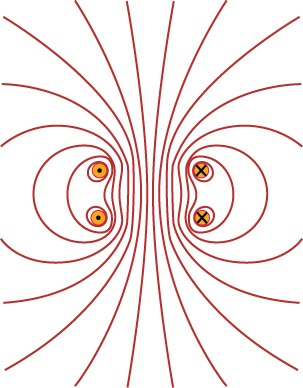Where is the field strength the greatest and in what direction do the magnetic field lines point?
1. The magnetic field strength is greatest where the magnetic field lines are less dense; magnetic field lines points up the page.
2. The magnetic field strength is greatest where the magnetic field lines are most dense; magnetic field lines points up the page.
3. The magnetic field strength is greatest where the magnetic field lines are most dense; magnetic field lines points down the page.
4. The magnetic field strength is greatest where the magnetic field lines are less dense; magnetic field lines points down the page.
30. The forces shown below are exerted on an electron as it moves through the magnetic field. In each case, what direction does the electron move?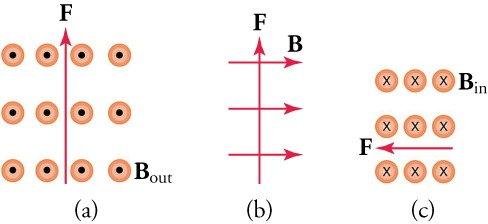1. (a) left to right, (b) out of the page, (c) upwards
2. (a) left to right, (b) into the page, (c) downwards
3. (a) right to left, (b) out of the page, (c) upwards
4. (a) right to left, (b) into the page, (c) downwards

#### 20.2Electromagnetic Induction

31. When you insert a copper ring between the poles of two bar magnets as shown in the figure, do the magnets exert an attractive or repulsive force on the ring? Explain your reasoning.
Figure 20.45
1. Magnets exert an attractive force, because magnetic field due to induced current is repulsed by the magnetic field of the magnets.
2. Magnets exert an attractive force, because magnetic field due to induced current is attracted by the magnetic field of the magnets.
3. Magnets exert a repulsive force, because magnetic field due to induced current is repulsed by the magnetic field of the magnets.
4. Magnets exert a repulsive force, because magnetic field due to induced current is attracted by the magnetic field of the magnets.
32.

The figure shows a uniform magnetic field passing through a closed wire circuit. The wire circuit rotates at an angular frequency of ω about the axis shown by the dotted line in the figure.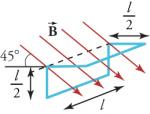What is an expression for the magnetic flux through the circuit as a function of time?

1. expression for the magnetic flux through the circuit Φ(t) = BAcos⁡ωt
2. expression for the magnetic flux through the circuit $Φ(t)=2BAcosωtΦ(t)=2BAcosωt$
3. expression for the magnetic flux through the circuit $Φ(t)=3BAcosωtΦ(t)=3BAcosωt$
4. expression for the magnetic flux through the circuit Φ(t) = 2BA cos ωt

#### 20.3Motors, Generators, and Transformers

33.
Explain why increasing the frequency of rotation of the coils in an electrical generator increases the output emf.
1. The induced emf is proportional to the rate of change of magnetic flux with respect to distance.
2. The induced emf is inversely proportional to the rate of change of magnetic flux with respect to distance.
3. The induced emf is inversely proportional to the rate of change of magnetic flux with respect to time.
4. The induced emf is proportional to the rate of change of magnetic flux with respect to time.
34.

Your friend tells you that power lines must carry a maximum current because P = I2R, where R is the resistance of the transmission line. What do you tell her?

1. Ptransmitted = Itransmitted2Rwire and Plost = ItransmittedVtransmitted, so I must be high to reduce power lost due to transmission.
2. Plost = Itransmitted2Rwire and Plost = ItransmittedVtransmitted, so I must be high to reduce power lost due to transmission.
3. Ptransmitted = Itransmitted2Rwire and Plost = ItransmittedVtransmitted, so I must be low to reduce power lost due to transmission.
4. Plost = Itransmitted2Rwire and Plost = ItransmittedVtransmitted, so I must be low to reduce power lost due to transmission.